# Java的作用

1. 首先学习JAVA语言
1. 基础语法
2. 面向对象
3. 常用类
4. 集合、线程、网络
2. Java web学习
1. Mysql
1. 配置
2. 安装
3. SQL
4. 数据库设计
2. 数据库编程
1. JDBC
2. 工厂模式
3. DAO/DTO
4. 数据库连接池
3. 网页设计
1. HTML
2. CSS
3. JAVASCRIPT
4. 框架
5. layui/bootstrap/jQuery
4. 交互
1. servlet
1. 创建与配置。。。
2. 待补充
2. JSP(Javaserverpage)--基于服务器运行
1. 服务器与HTTP协议
5. 项目实战 还需要补充以及更新

# 第一部分 JAVA学习

## 名词解释

• JVM
虚拟机，模逆运行环境
• JRE 不做开发，只运行，JRE足够 运行环境，包含JVM和解释器，完整的运行环境
• JDK 需要进行开发活动 包含JRE+类库+开发工具包（编译器+调试工具）

## 安装

### 配置环境变量

1. 编辑系统环境变量，
2. 新建系统环境变量
3. 新建%JAVA_HOME%变量，路径为java根目录
4. 编辑系统变量中的path，新建路径%JAVA_HOME%\bin
5. 打开cmd，使用java -version和javac命令检测是否成功。

## 开始java

java的文件的演变过程：.java -->.class-->解释器运行

### helloworld

``````class helloworld {
public static void main(String[] args){
System.out.print("HEllo world!")；
}
}
``````
• helloworld类，作为容器
• main为方法，即C语言中的main函数入口。

CMD中调用javac指令编译java文件，生成**class（字节码）**文件。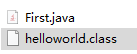两个文件

### 关于报错以及代码规范

• 规范： 在声明类的时候，如果用public声明类，那么文件名必须跟public声明的类名一样。同理，在一个类文件中声明了一个public类后，其余的类不允许声明为public。

• 规范： java对大小写敏感，注意大小写以及中英文符号。

• 规范： 类名首字母最好大写。

-encoding utf-8

## 变量

### 命名规则：

• 可以由数字、字母、_、货币符号组成，数字不能作为开头（同C）
• 不能与关键字与保留字重名
• 不能与字面常量重名，比如true、false、null。
• 驼峰命名
• 类用首字母大写
• 变量名用驼峰，首个单词开头小写，第二个开头大写，以此类推。

### 数据类型

java中有两种数据类型，即基本数据类型引用数据类型

#### 基本数据类型

1. 整数
大小 整数类型
1字节 byte
2字节 short
4字节 int
8字节 long
2. 浮点数（java默认浮点数为double类型，若要用float，在小数后面加f）
大小 浮点数类型 精度
4字节 float 单精度
8字节 double 双精度
3. boolean 4字节 真与假

1. 字符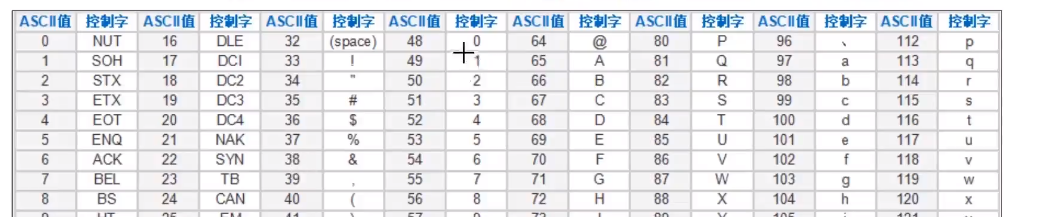主要关注0、A和a即可。 char类型，占用2字节，采用Unicode编码。赋值同C，如

``````char = 'A'; char = 48; char ='\u0041';
``````

#### 引用数据类型

1. 字符串String

``````String str1="hello";
``````
2. 数组

待补充

## CMD交互

• .nextInt();//获得整数
• .nextDouble();//获得小数
• .next();//获得字符串
• .next().charAt(0);//获得单个字符，即字符串中的第0个位置的字符
``````import java.util.Scanner;
public class variable{
public static void main(String[] args){
Scanner input=new Scanner(System.in);
System.out.println("Input ID:");
String name= input.next();
}
}
``````这就是上述代码的结果。

PS:本节开始体现出与C的区别，调用类必须要给ta个容器，如input这里。

``````import java.util.Scanner;
public class trans2Unicode{
public static void main(String[] args){
System.out.println("Input a character输入单字符: ");
Scanner input=new Scanner(System.in);
char t1 = '中';
String t2 = input.next();
System.out.println((int)t1);
System.out.println(t2);
}
}
``````

``````import java.util.Scanner;
public class trans2Unicode{
public static void main(String[] args){
System.out.println("Input a character输入单字符: ");
Scanner input=new Scanner(System.in,"GBK");
char t1 = '中';
String t2 = input.next();
System.out.println((int)t1);
System.out.println(t2);
}
}
``````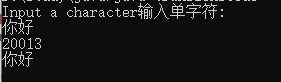这是GBK编码。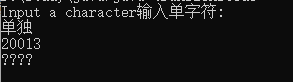这是utf-8编码。

``````import java.util.Scanner;
public class test2unicode{
public static void main(String[] args){
System.out.println("输入:");
Scanner input= new Scanner(System.in);
char _id=input.next().charAt(0);
System.out.println(_id+"的编码为："+(int)_id);
}
}
``````

### 循环

``````public class Main {
public static void main(String[] args) {
for(int i=1;i<6;i++){
for(int j=1;j<=i;j++)
System.out.print("*");
System.out.println("");
}
}
}
``````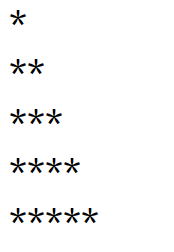输出如图所示。

PS:已经不再使用CMD进行源文件编译，使用IDEA编译，相关配置与该教程相近

1. 对于java来说，好像每个类都可以有一个main函数，这与C语言有着很大的不同，经过查询，貌似只会调用主类的main作为入口。 2. 包的概念貌似只是用来方便管理。 3. 包的名称必须用域名的倒序来书写。 4. 类的概念较为抽象，一系列的运算可以以类为单位

``````public class calendarTest {
public static void main(String[] args){
//输入日期
Scanner inputDate = new Scanner(System.in);
System.out.println("请输入年份(1900年之后）：");
int year=inputDate.nextInt();
System.out.println("请输入月份: ");
int month=inputDate.nextInt();
//判断年月的合法性-
//计算年份月份的对应
int daysum=0;
//平年闰年
for(int i=1900;i<year;i++){
if (i%4==0 && i%100!=0 || i%400 ==0)
daysum+=366;
else daysum+=365;
}
for(int i = 1 ;i<month;i++){
if(i==1 || i==3 || i==5 || i==7 || i==8 || i==10 || i==12)
daysum+=31;
else if(i==4||i==6||i==9||i==11)
daysum+=30;
else if(i==2){
if(year%4==0 && year%100!=0 || year%400 ==0)
daysum+=29;
else daysum+=28;
}
else daysum+=28;
}
int weekDate = 1+daysum%7;
if(month==1 || month==3 || month==5 || month==7 || month==8 || month==10 || month==12)
daysum=31;
else if(month==4||month==6||month==9||month==11)
daysum=30;
else if(month==2){
if(year%4==0 && year%100!=0 || year%400 ==0)
daysum=29;
else daysum=28;
}
month=1;
while (daysum>0){
System.out.print(month+"号为");
switch (weekDate)
{
case 1:System.out.print("星期一\n");break;
case 2:System.out.print("星期二\n");break;
case 3:System.out.print("星期三\n");break;
case 4:System.out.print("星期四\n");break;
case 5:System.out.print("星期五\n");break;
case 6:System.out.print("星期六\n");break;
case 0:System.out.print("星期天\n");break;
}
weekDate=(weekDate+1)%7;
month++;
daysum--;
}
}
}
``````

``````month=1;
System.out.print("一\t二\t三\t四\t五\t六\t日\n");
for(int i=weekDate-1;i>0;i--)
System.out.print("\t");
while (daysum>0){
System.out.print(month+"\t");
weekDate=weekDate%7;
if (weekDate==0)
System.out.print("\n");
weekDate++;
month++;
daysum--;
}
``````

## 方法

``````public static void name(){
}
``````

void返回值类型，同C一样。方法的定义要在类中，与main方法平级，这里与C不同。实参与形参同C。

## 数组

``````int[] a=new int;
//或者
int[] a;
a=new int;
``````

``````//带有初始值的,只能在声明的时候初始化，上述第二种声明方法无法初始化。
int[] a ={1,2,3,4,5};
//or
int[] a=new int[]{1,2,3,4,5};
``````

java中的数组有个.length属性，可以直接输出长度。

### 数组扩容

1. 创建一个大于原数组长度的数组
2. 旧数组复制到新数组

1. System.arraycopy(原数组，原数组起始，新数组，新数组起始);
2. java.util.Arrays.copyOf(原数组，新长度);//返回带有原值的新数组 注意上述的返回值描述，必须要用个地址接收，如下：
`````` int[] a={1,2,3,4,5};
a=Arrays.copyOf(a,a.length*2);
System.out.println(Arrays.toString(a));
``````
如果没有接收，如下：
`````` int[] a={1,2,3,4,5};
Arrays.copyOf(a,a.length*2);
System.out.println(Arrays.toString(a));

``````

### 可变长参数

``````add ( int ... nums){
...
}
``````

### 排序

``````  public static int[] bubbleSort(int[] nums){
int temp;
for (int i=0;i<nums.length-1;i++) {
for (int j = 0; j < nums.length - i - 1; j++) {
if (nums[j] > nums[j + 1]) {
temp = nums[j];
nums[j] = nums[j + 1];
nums[j + 1] = temp;
}
}
}
return nums;
}
``````

java中自带排序方法，Arrays.sort()（升序）。 计数排序很有意思。

### 查找

• 顺序查找
• 二分查找，Arrays.binarySearch(nums,num)自带的二分查找

## 面向对象

### 类的概念

“类”就是模板，蓝图，比如一辆汽车，它有着一个设计图，设计图就是类。 而“对象”就是根据这张蓝图new出来的一辆具体的汽车，也就是前文中的“容器”。

``````public class Hero {
//属性
String name;
String position;
int attack;
int prevent;
//行为
public void eat(){
System.out.println(name+"在吃药");
}
public void move(){
System.out.println(name+"在赶路");
}
}
``````

### 方法重载

``````public void eat (int a){
}
public void eat (string a){
}
``````

### 构造方法

• 用于创建对象的方法。
• 没有返回值类型
• 名称与类名相同
• 只能通过new调用，不能用.**来调用

``````public eat(int a,int b){
}
public eat(int a){
this(a,b)//必须作为第一行语句执行
}
``````

## 封装

``````setAge(int age){
this.age=age;
}
getAge(){
return this.age;
}
``````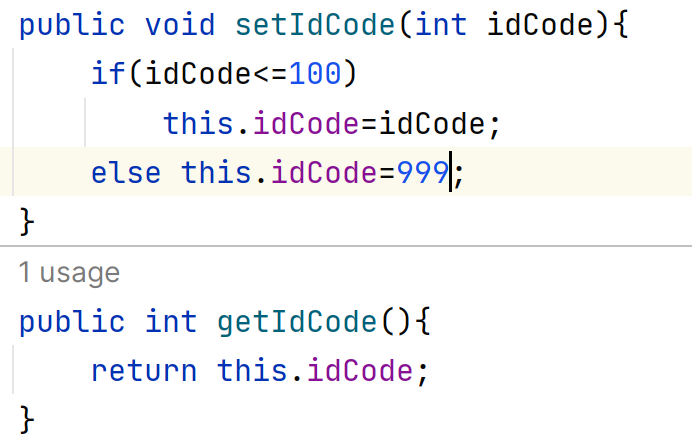封装的好处还有方便修改，比如修改了类中的变量名，没有封装的话要全部修改，有封装的话只需要修改类中的变量名就可以。

## 继承

1. 属性：名
2. 属性：健康值
3. 属性：品种
4. 方法：跑
5. 方法：吃
1. 企鹅
1. 属性：名
2. 属性：健康值
3. 属性：性别
4. 方法：游
5. 方法：吃
1. 属性：名
2. 属性：健康值
3. 属性：亲密度
4. 属性：品种
5. 方法：飞
6. 方法：吃

1. 属性：名
2. 属性：健康值
3. 方法：吃

### 使用方法

``````public class *** extends *** {
...
}//在类声明加入 extends + 父类名称
``````

### 继承的特点

#### 属性的继承

1. 父类所有属性都可以被继承
2. 父类的私有属性能被继承但不能访问
3. 如果子类定义了与父类相同的变量名，优先从最底层（子类）开始找。同名属性可以通过super关键字进行区分 （在实际测试中我发现父类的同名变量会优先使用，跟课程中不一样）
4. 当子类的成员变量与父类同名时，若对该成员变量进行操作的方法继承于父类，则改变和获取的是父类的成员变量，父类属性不可被重写,只会被调用,父类方法可以被重写,也可以被调用
``````class 父类(){
int b;
}
class 子类 extends(){
int b;
super.b=3;
this.b=2;
}
``````

## 访问修饰符

private-本类中访问 public-项目中的任何地方都可以访问 default-作用于本类中或者同包中 protected-本类、本包、其他包中的子类

## 方法的重写/覆盖

• 重写/覆盖
• 当父类的方法不能满足子类需求时，需要方法重写
• 访问权限可以放大，比如父类方法为protected，子类重写方法为public
• 返回值类型可以跟父类一致，也可以是父类返回类型的子类
• 方法名一定要一样
• 传参要一样，否则为方法重载了

##继承中的对象创建 在具有继承关系的对象的创建中，构建子类对象会优先调用父类构造。 但是并没有构造父类的空间，而是在子类对象的属性空间里。

# 多态

``````Pet pet=new Dog();
``````

### 向上转型

Pet与Dog必须具有直接或间接的继承关系。但是只能调用父类中的公用方法，执行子类的方法——重写作为基础。

## 多态的应用

1. 父类类型作为形参
2. 父类类型作为返回值实现多态

### 向下转型

``````Pet pet = new Dog();
Dog dog = (Dog)pet;
``````

## 抽象类abstract

### 抽象方法

``````//抽象方法只需要声明，不需要实现
public abstract void eat();
``````

## 静态static

• Arrays.copyOf()
• Arrays.sort()
• Math.random()
• Math.sqrt
• 使用类名调用 静态方法可以继承，不能重写，没有多态。也就是说只用类有关，与对象是谁无关。 静态方法类似重写的操作成为隐藏。 静态属性只能定义在类中，不能在方法中！

### 静态代码块

``````static{

}
``````

final关键字：

• 修饰类，类不能继承
• 修饰方法，方法不能重写
• 修饰变量，就是常量

# 接口

``````//类
class dog(){
}
//接口
interface dog(){
public final static int m;
public final static void m1();
}
``````

JDK8以前接口中只能写公开静态常量，公开静态方法。

``````//调用接口
public class subtest impements dog{
//重写方法
}
``````

## 什么是接口？

``````public class student implements imusic,icode{
...
}
``````

## 接口标准

``````public interface Iusb {
void service_();
}//定义接口
public class Usbdisk implements Iusb{
public void service_(){
System.out.println("使用U盘");
}
}//接口的实现1
public class Usbfan implements Iusb{
public void  service_(){
System.out.println("使用USB风扇");
}
}//接口的实现2
public class Computer {
Iusb usb;
}//包含接口标准的类
public class Test {
public static void main(String[] args){
Computer com1 = new Computer();
Usbdisk ud=new Usbdisk();
Usbfan uf=new Usbfan();
com1.usb=ud;
com1.usb.service_();
}
``````

## 接口回调

``````public interface ICall(){
void call();
default doCall(){
call();
}
}
``````

（难以理解） #Object类的方法 任何类都直接或者间接继承了object类的方法。

# 内部类

``````//Outer外部类 In内部类
Outer out=new Outer();
In in= out.new In();
in.inmethod();
``````

``````
In in=new Outer().new In();
``````

``````class Out {
int num=1;
class in{
int num=2;
void inMethod(Out out){
out.num++;
}
}
}
``````

``````class Out {
int num=1;
class in{
int num=2;
void inMethod(){
out.this.num++;
}
}
}
``````

### 匿名内部类

``````public interface IUsb {
void service();
}
public abstract class Sup {
public abstract void test();
}

``````
``````public class Noname {
public static void main(String[] args){
IUsb usbdisk=new IUsb() {
public void service(){
System.out.println("使用U盘");
}
};
usbdisk.service();

Sup sub=new Sup(){
public void test(){
System.out.println("抽象父类");
}
};
sub.test();
}
}
}
``````

byte Byte
short Short
int Integer
long Long
float Float
double Double
char Character
boolean Boolean

###装箱、拆箱

# 正则表达式

• ^表示正则表达式开头，\$表示结尾
• \d表示数字，\d{6}表示匹配6位数字,\d{6,10}6到10位
• 两种检测方法：

#Random类

``````Random r=new Random(种子);
r.nextInt(规定最高为多少)
``````